Wiedemann-Franz Law

Also found in: Wikipedia.

Wiedemann-Franz law

An empirical law of physics which states that the ratio of the thermal conductivity of a metal to its electrical conductivity is a constant times the absolute temperature, as given by the equation below. Here Kc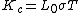is the thermal conductivity due to the conduction electrons, σ is the electrical conductivity, T is the absolute temperature, and L0 is known as the Lorentz number. The Wiedemann-Franz law provides an important check on theories of electrical and thermal conductivity. See Conduction (heat), Thermal conduction in solids

McGraw-Hill Concise Encyclopedia of Physics. © 2002 by The McGraw-Hill Companies, Inc.
The following article is from The Great Soviet Encyclopedia (1979). It might be outdated or ideologically biased.

Wiedemann-Franz Law

the correlation between the heat conductivity and electrical conductivity of metals.

In 1853, G. Wiedemann and R. Franz (1827-1902) established on the basis of experimental data that for all metals the ratio of heat conductivity K to electrical conductivity σ at a constant temperature is the same: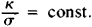In 1882 the Danish physicist L. Lorenz demonstrated that the relationship k/σ changes in direct proportion to the abso-lute temperature T: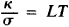where L is a constant that is identical for all metals; this is called the Lorenz number.

The interconnection between electrical conductivity and heat conductivity is explained by the fact that both these properties of metals are mainly caused by the movement of electrons. The Wiedemann-Franz law was explained for the first time by the German physicist P. Drude, who regarded electrons in metal as a gas and who applied to them the methods of the classical kinetic theory of gases. However, it was only with the aid of quantum statistics that an expression was obtained for L that was in good agreement with the experiment: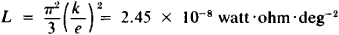where k is the Boltzmann constant and e is the charge of an electron. However, the Wiedemann-Franz law is consistent with experiment only within a fixed interval of temperatures (see Figure 1).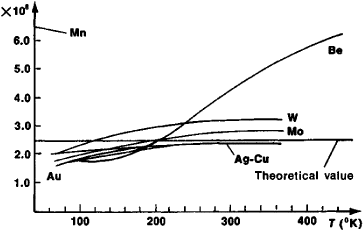Figure 1. Dependence of the Lorenz number, L X 108, on temperature T for certain metals

Present-day solid-state theory, which takes into consideration the interaction of electrons with crystal-lattice vibrations (electron-phonon interaction), explains not only the Wiedemann-Franz law but also the cause of deviations from it. At room temperature the observed values of L are fully consistent with its theoretical values. However, there are metals (for example, Be), which at room temperature are not subject to the Wiedemann-Franz law. The reason for this is apparently the large value of the Debye temperature for these metals.

Wiedemann-Franz law

[′vēd·ə‚män ′fränts ‚lȯ]
(solid-state physics)
The law that the ratio of the thermal conductivity of a metal to its electrical conductivity is a constant, independent of the metal, times the absolute temperature. Also known as Lorentz relation.
McGraw-Hill Dictionary of Scientific & Technical Terms, 6E, Copyright © 2003 by The McGraw-Hill Companies, Inc.
References in periodicals archive ?
Recently, hexagonal Boron Nitride (h-BN) bottom substrate can be a great heat drained material for disordered graphene so as to extremely reduce the carrier-phonon scattering via Wiedemann-Franz law heat diffusion, which is [delta] = 2 [15-17].
Based on the self-thermometer linear properties between [T.sub.e] and I due to Wiedemann-Franz law heat diffusion, we can investigate energy relaxation cooling processes under high carrier density limit ([T.sub.BG] > [T.sub.L]) between the h-BN/CVD graphene and multilayer exfoliated graphene for future graphene-based applications.
Particularly, [beta] [approximately equal to] 2, the heat diffusion described by the Wiedemann-Franz law, was found in graphene on bottom h-BN substrate systems, where bottom h-BN substrate acts as a thermal conduction layer, effectively reducing the electron-phonon coupling [14-16].
But in the recent study, titled "Anomalously low electronic thermal conductivity in metallic vanadium dioxide," it was found that the material exhibited properties that are exceptions&nbsp;to the Wiedemann-Franz Law which establishes&nbsp;a general relationship between electrical and thermal conductivity.
Using results from simulations and X-ray scattering experiments, scientists at the Department of Energy's Lawrence Berkeley National Laboratory (Berkeley Lab) and at the University of California, Berkeley, found the electrons in vanadium dioxide are able to conduct electricity without conducting heat at a rate of thermal conductivity which was "ten times smaller than what would be expected from the Wiedemann-Franz Law."
Four-probe electrical resistivity ([rho]) was measured simultaneously using the same gold wires for voltage measurement, allowing a direct verification of the setup using the Wiedemann-Franz law ([kappa][rho]/T [right arrow] [L.sub.0] for T [right arrow] 0 with [L.sub.0] = 2.44 x [10.sup.-8] W[OMEGA][K.sup.-2]).
For T [right arrow] 0 the Wiedemann-Franz law L = [L.sub.0] is obeyed within 5% which reflect the validity of our measurements.
Then we calculated the electronic contribution to thermal conductivity using the Wiedemann-Franz law: [[kappa].sub.N]/T = [L.sub.0]/[rho].
Their experiments on purple bronze, a metal with unique one-dimensional electronic properties, indicate that it breaks the Wiedemann-Franz Law.
For the past 150-plus years, the Wiedemann-Franz law has proved to be remarkably robust, the ratio varying at most by around 50 per cent amongst the thousands of metallic systems studied.
The lattice contribution to the thermal conductivity was dominant over the carrier contribution for [Mg.sub.2+x][Si.sub.0.7][Sn.sub.0.3][Sb.sub.m] specimens because their thermal conduction behavior was inconsistent with the Wiedemann-Franz law .
In order to clarify the phonon scattering effect by pores we calculated the [[kappa].sub.tot] using the following equation (inset of Figure 5): [[kappa].sub.lat] = [[kappa].sub.tot] - [[kappa].sub.ele], where the electronic contribution ([[kappa].sub.ele]) is estimated from the Wiedemann-Franz law, [[kappa].sub.ele] = [L.sub.0] [[sigma].sup.T], with the Lorentz number [L.sub.0] = 2 x [10.sup.-8] W [OMEGA] [K.sup.-2].

Site: Follow: Share:
Open / Close